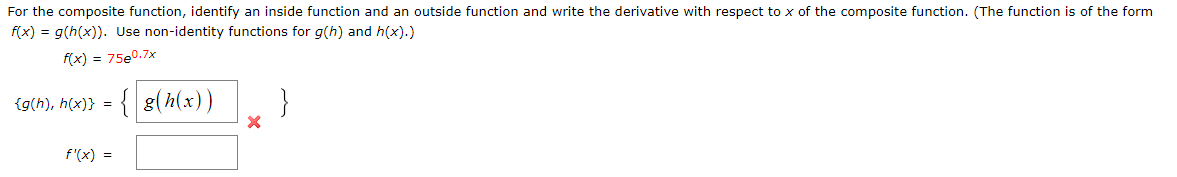# For the composite function, identify an inside function and an outside function and write the derivative with respect to x of the composite function. (The function is of the formg(h(x)). Use non-identity functions for g(h) and h(x).)f(x)f(x) 75e0.7x}g(h), h(x)) = {g(h(x))f'(x)

Question
1 viewshelp_outlineImage TranscriptioncloseFor the composite function, identify an inside function and an outside function and write the derivative with respect to x of the composite function. (The function is of the form g(h(x)). Use non-identity functions for g(h) and h(x).) f(x) f(x) 75e0.7x } g(h), h(x)) = {g(h(x)) f'(x) fullscreen
check_circle

Step 1

The given funct...

### Want to see the full answer?

See Solution

#### Want to see this answer and more?

Solutions are written by subject experts who are available 24/7. Questions are typically answered within 1 hour.*

See Solution
*Response times may vary by subject and question.
Tagged in

### Other